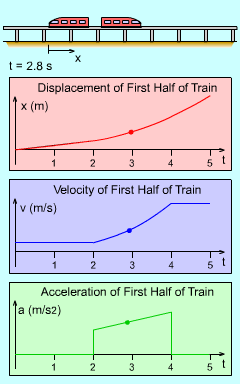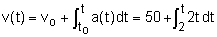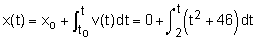Ch 1. Particle General Motion Multimedia Engineering Dynamics Position,Vel & Accel. Accel. varyw/ Time Accel. Constant Rect. Coordinates Norm/Tang. Coordinates Polar Coordinates RelativeMotion
 Chapter - Particle - 1. General Motion 2. Force & Accel. 3. Energy 4. Momentum - Rigid Body - 5. General Motion 6. Force & Accel. 7. Energy 8. Momentum 9. 3-D Motion 10. Vibrations Appendix Basic Math Units Basic Equations Sections Search eBooks Dynamics Fluids Math Mechanics Statics Thermodynamics Author(s): Kurt Gramoll ©Kurt GramollDYNAMICS - CASE STUDY SOLUTIONTrain Motion When integrating the acceleration to determine the velocity and position, the initial conditions must be specified. The lower limits of integration will be      vo = 50 m/s      to = 2 s When the acceleration is integrated, the expression for the velocity, as a function of time, becomes= t2 + 46      v(t = 4) = 62 m/s The next step is to integrate the velocity. However, it is important that v(t) is integrated and not the velocity at 2 seconds, 62 m/s. This is a common mistake. Integrating the velocity function, v(t), gives an expression for the position as a function of time,= 0.333 t3 + 46 t - 94.67      x(t = 4) = 110.7 m Note that the initial position xo was set to zero when to = 2 so that the equation for x(t) gives the change in position starting at t = 2.

Practice Homework and Test problems now available in the 'Eng Dynamics' mobile app
Includes over 400 problems with complete detailed solutions.
Available now at the Google Play Store and Apple App Store.